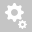##Recently viewed tickets• Sales
• Library Model Request
• Technical Support

# Why is the TMS result differnt between using series resistance and Liquid Series Resistance when data input are same?

Two cases:
Motor and load model are the same. Torque, current and moment of inertia are also the same.

One case has series resistance start and the other has liquid resistance start.

The starters types have same input data as well. IE, the corresponding Resistance and Speed are the same for both cases.

However, after running TMS the result does not look to be the same.

The reason is because Liquid Resistance Starter uses a linear interpolation continuous curve for resistance and speed (pu), while series Resistance used 3 discrete points.

That is for Liquid Resistance Starter, the corresponding resistance value that the software uses between Speed (pu) points is interpolated.
For Resistance Starter, the corresponding resistance value between Speed (pu) points is not interpolated. The higher impedance value is used between Speed (pu) points.